Examples

Chapter 2 Class 11 Relations and Functions
Serial order wise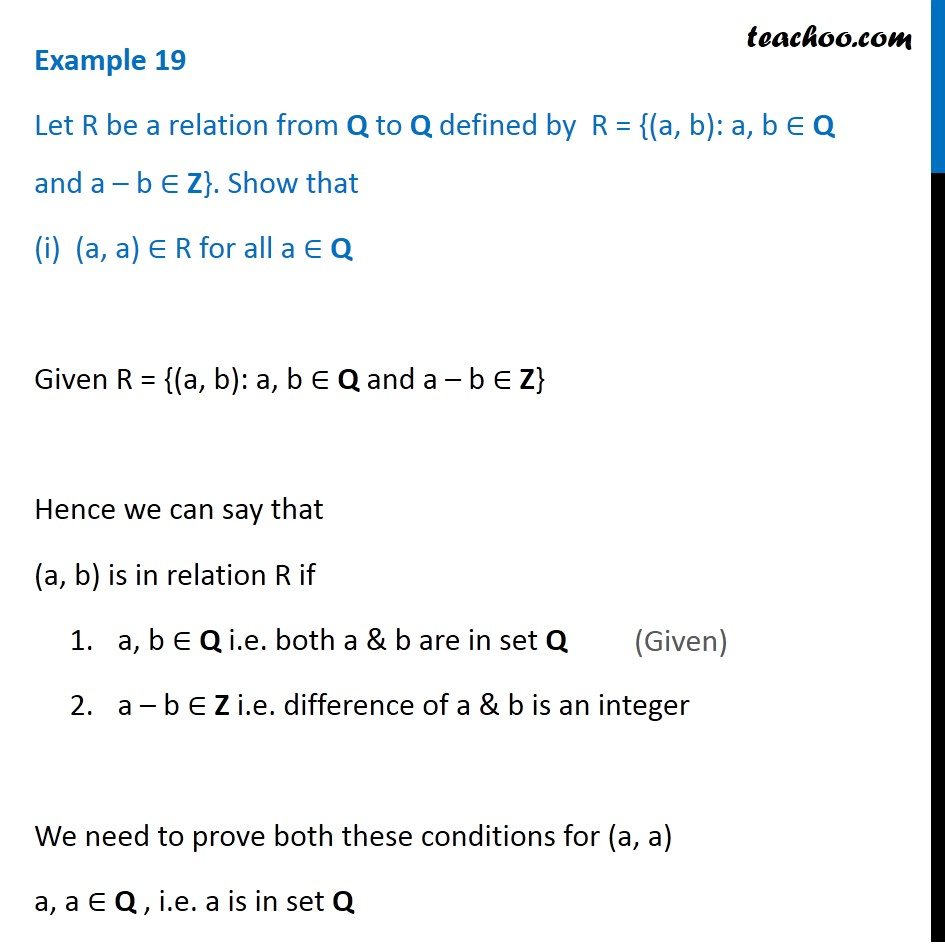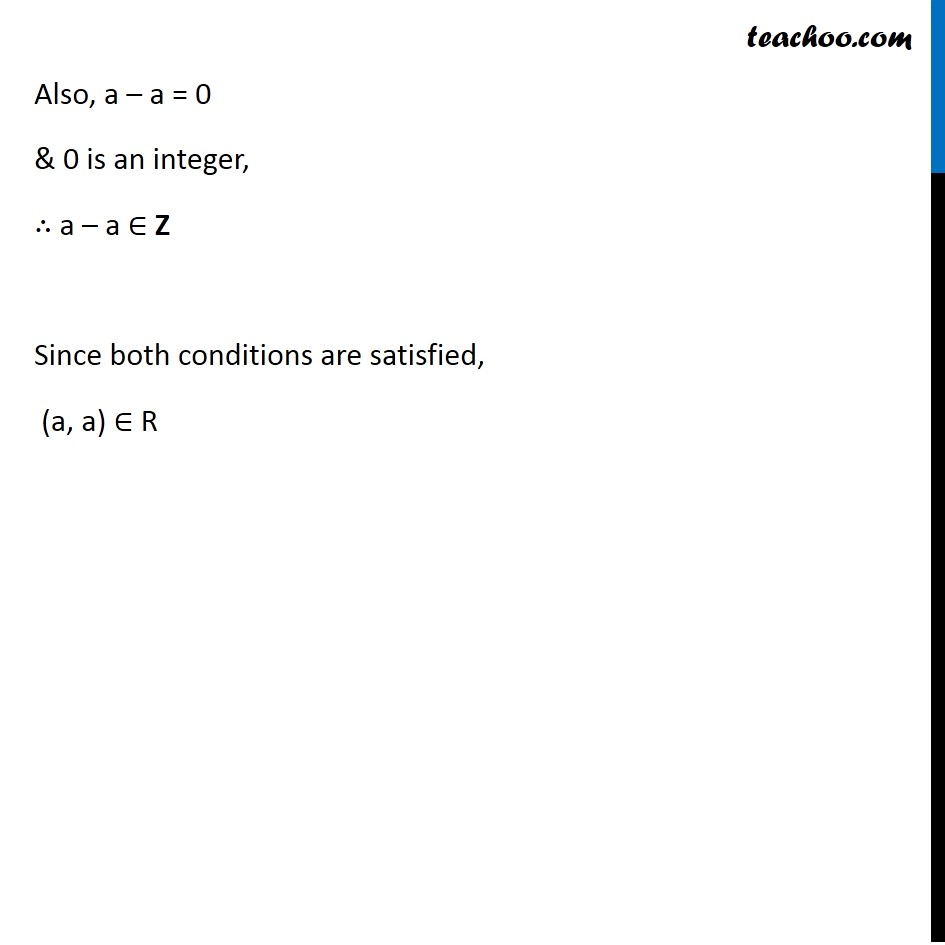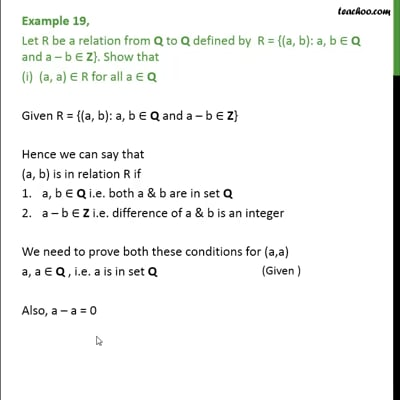This video is only available for Teachoo black users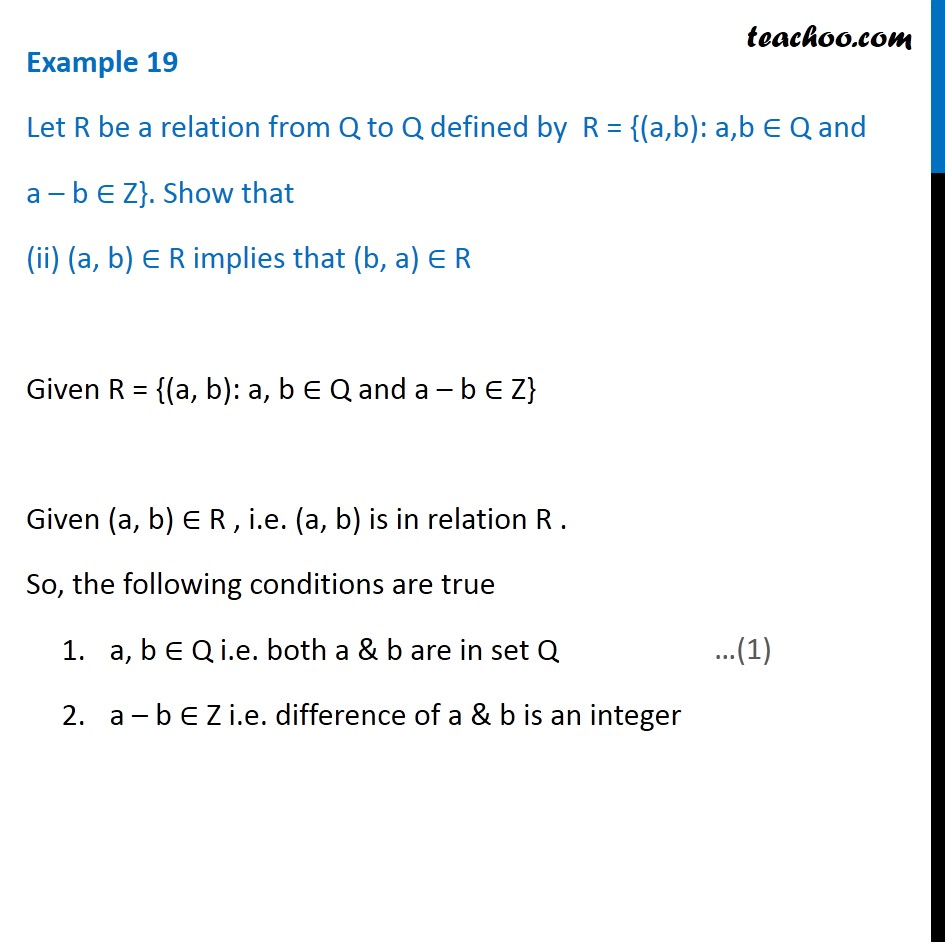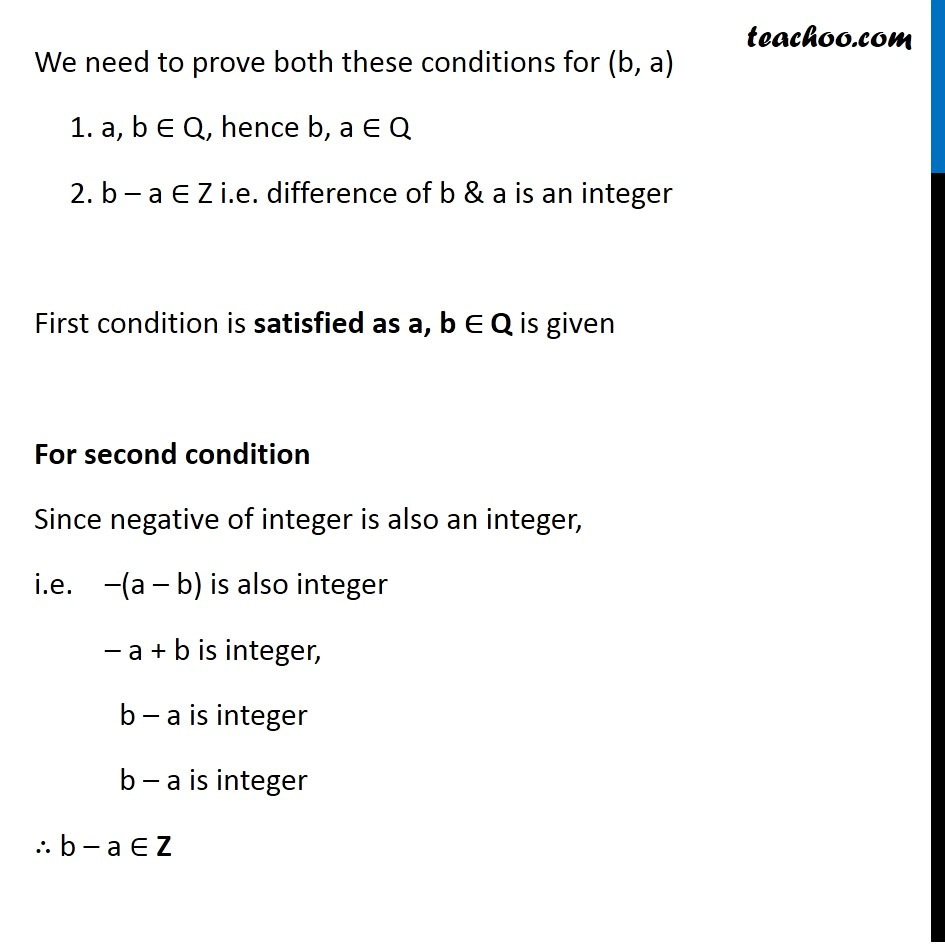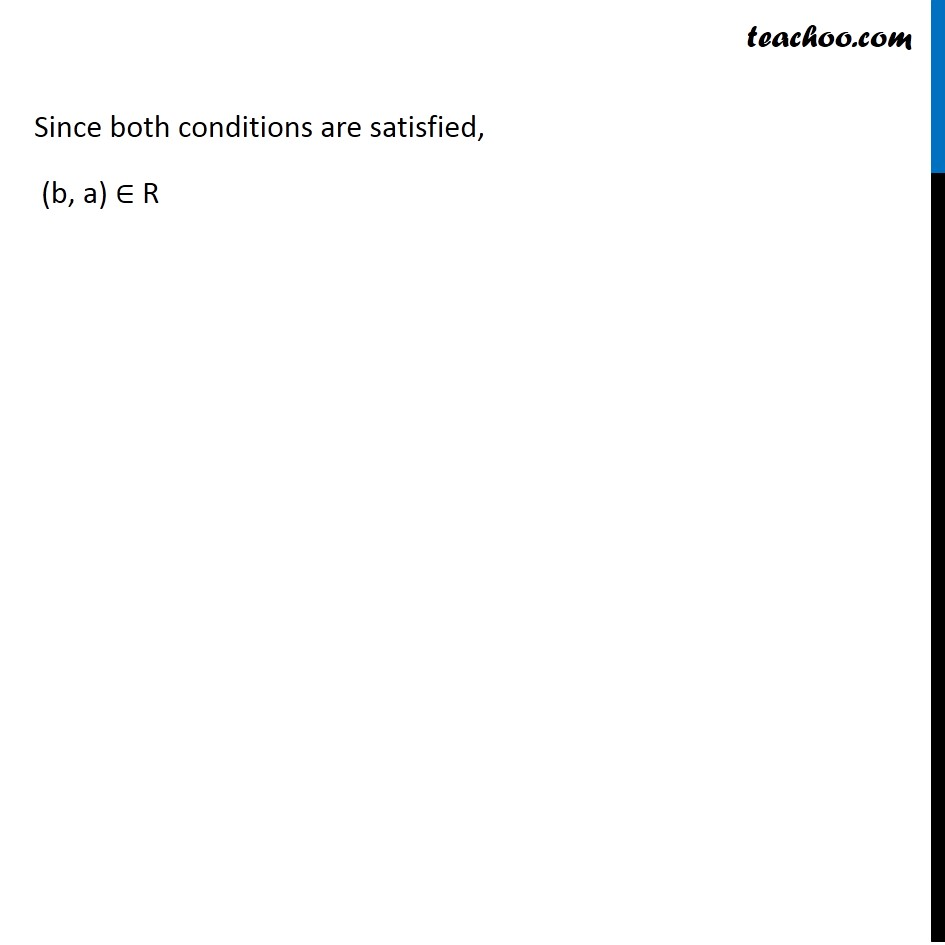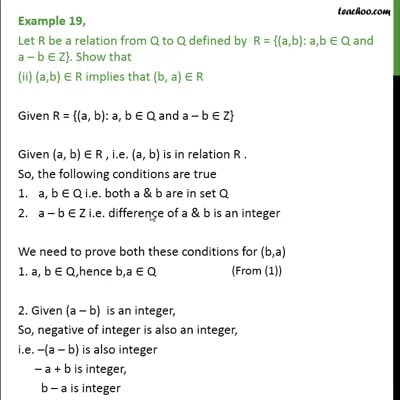This video is only available for Teachoo black users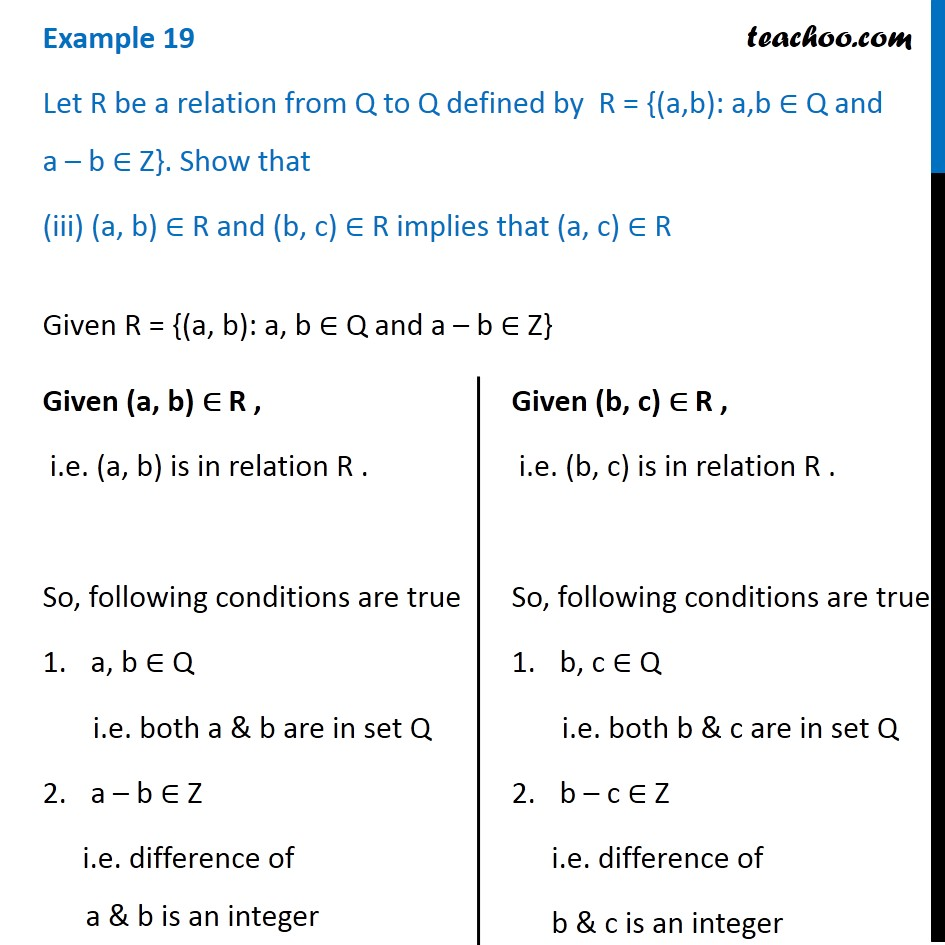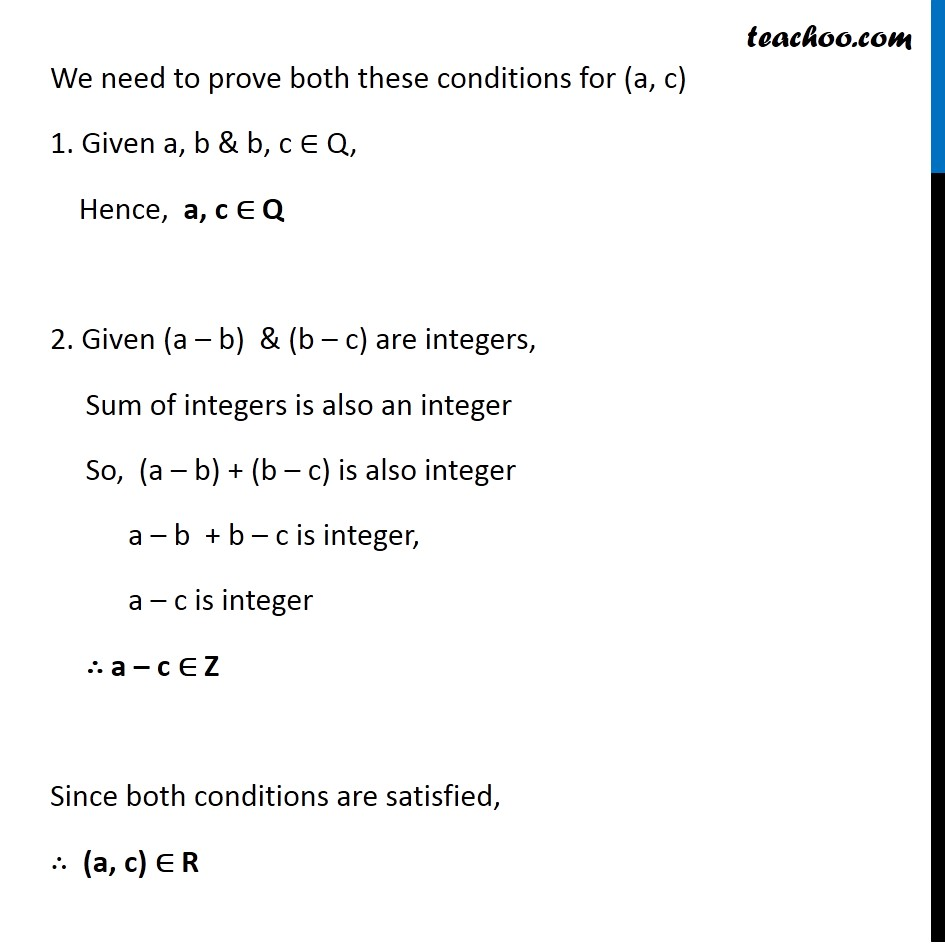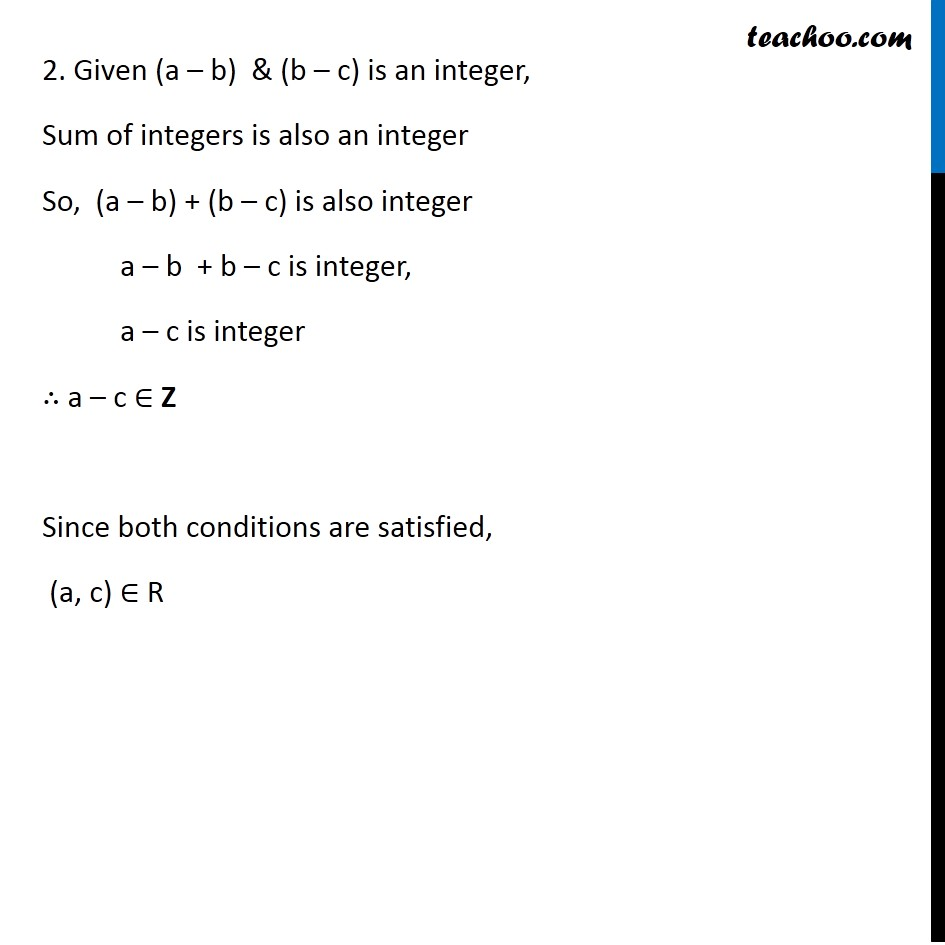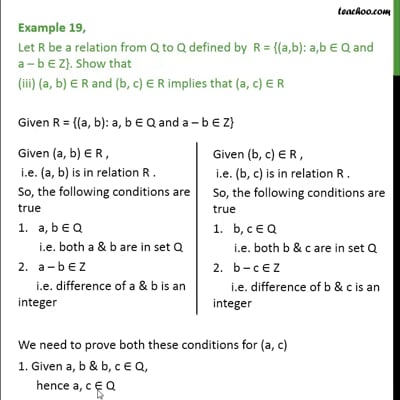This video is only available for Teachoo black users

Maths Crash Course - Live lectures + all videos + Real time Doubt solving!

### Transcript

Example 19 Let R be a relation from Q to Q defined by R = {(a, b): a, b ∈ Q and a – b ∈ Z}. Show that (i) (a, a) ∈ R for all a ∈ Q Given R = {(a, b): a, b ∈ Q and a – b ∈ Z} Hence we can say that (a, b) is in relation R if a, b ∈ Q i.e. both a & b are in set Q a – b ∈ Z i.e. difference of a & b is an integer We need to prove both these conditions for (a, a) a, a ∈ Q , i.e. a is in set Q Example 19 Let R be a relation from Q to Q defined by R = {(a, b): a, b ∈ Q and a – b ∈ Z}. Show that (i) (a, a) ∈ R for all a ∈ Q Given R = {(a, b): a, b ∈ Q and a – b ∈ Z} Hence we can say that (a, b) is in relation R if a, b ∈ Q i.e. both a & b are in set Q a – b ∈ Z i.e. difference of a & b is an integer We need to prove both these conditions for (a, a) a, a ∈ Q , i.e. a is in set Q Also, a – a = 0 & 0 is an integer, ∴ a – a ∈ Z Since both conditions are satisfied, (a, a) ∈ R Example 19 Let R be a relation from Q to Q defined by R = {(a,b): a,b ∈ Q and a – b ∈ Z}. Show that (ii) (a, b) ∈ R implies that (b, a) ∈ R Given R = {(a, b): a, b ∈ Q and a – b ∈ Z} Given (a, b) ∈ R , i.e. (a, b) is in relation R . So, the following conditions are true a, b ∈ Q i.e. both a & b are in set Q a – b ∈ Z i.e. difference of a & b is an integer We need to prove both these conditions for (b, a) 1. a, b ∈ Q, hence b, a ∈ Q 2. b – a ∈ Z i.e. difference of b & a is an integer First condition is satisfied as a, b ∈ Q is given For second condition Since negative of integer is also an integer, i.e. –(a – b) is also integer – a + b is integer, b – a is integer b – a is integer ∴ b – a ∈ Z Since both conditions are satisfied, (b, a) ∈ R Example 19 Let R be a relation from Q to Q defined by R = {(a,b): a,b ∈ Q and a – b ∈ Z}. Show that (iii) (a, b) ∈ R and (b, c) ∈ R implies that (a, c) ∈ R Given R = {(a, b): a, b ∈ Q and a – b ∈ Z} Given (a, b) ∈ R , i.e. (a, b) is in relation R . So, following conditions are true a, b ∈ Q i.e. both a & b are in set Q a – b ∈ Z i.e. difference of a & b is an integer Given (b, c) ∈ R , i.e. (b, c) is in relation R . So, following conditions are true b, c ∈ Q i.e. both b & c are in set Q b – c ∈ Z i.e. difference of b & c is an integer We need to prove both these conditions for (a, c) 1. Given a, b & b, c ∈ Q, Hence, a, c ∈ Q 2. Given (a – b) & (b – c) are integers, Sum of integers is also an integer So, (a – b) + (b – c) is also integer a – b + b – c is integer, a – c is integer ∴ a – c ∈ Z Since both conditions are satisfied, ∴ (a, c) ∈ R 2. Given (a – b) & (b – c) is an integer, Sum of integers is also an integer So, (a – b) + (b – c) is also integer a – b + b – c is integer, a – c is integer ∴ a – c ∈ Z Since both conditions are satisfied, (a, c) ∈ R